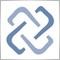Question about for loop iteration to optimize24

Just wondering if I define an int bar in the scope of OnCalculate and later I use a for loop such as for(bar=prev_calculated; bar<rates_total/units_per_bar; bar++) will bar retain the incremented value after iterations or is the bar variable in the for loop working in a different scope? I am assuming that I can use prev_calculated as the index value of the next unfilled bar based on the idea that prev_calculated - 1 is the index of the last bar with calculated data. Also I wanted to know if the variable like int is defined but not initialized, if it might contain 0 by the compiler or if memory may contain garbage from RAM like in c compiled code. Thanks, this info will help me optimize my imdicator. By the way I am on a mobile phone only until next month otherwise I would have written test code on a computer to observe behavior. I'm using hand written code for now.6659

forlorn_beast:
Just wondering if I define an int bar in the scope of OnCalculate and later I use a for loop such as for(bar=prev_calculated; bar<rates_total/units_per_bar; bar++) will bar retain the incremented value after iterations or is the bar variable in the for loop working in a different scope? I am assuming that I can use prev_calculated as the index value of the next unfilled bar based on the idea that prev_calculated - 1 is the index of the last bar with calculated data.

You can find answer to your question here (https://www.mql5.com/en/docs/basis/variables/variable_scope). If you define variable int bars before loop and don't redefine loop variable with the same name like this

int bars;
for(int bars=SOME_VALUE;bars<1000;bars++) { SOME ACTIONS... }

variable will retain incremented value after exiting loop.

Also I wanted to know if the variable like int is defined but not initialized, if it might contain 0 by the compiler or if memory may contain garbage from RAM like in c compiled code. Thanks, this info will help me optimize my imdicator.
Just read help topic https://www.mql5.com/en/docs/basis/variables/initialization
Documentation on MQL5: Language Basics / Variables / Visibility Scope and Lifetime of Variables
• www.mql5.com
Language Basics / Variables / Visibility Scope and Lifetime of Variables - Documentation on MQL5